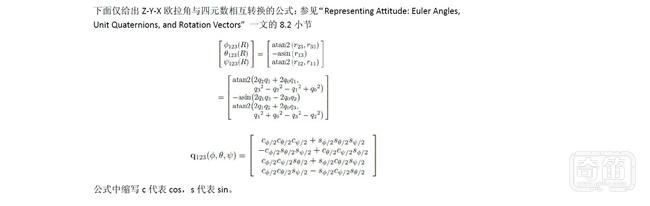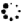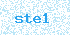﻿ 【挑挑软件的筋】：一种常见的四轴飞行器姿态解算方法分析 | 奇笛网

### 【挑挑软件的筋】：一种常见的四轴飞行器姿态解算方法分析Kp，Ki，控制加速度计修正陀螺仪积分姿态的速度
halfT ，姿态解算时间的一半。此处解算姿态速度为500HZ，因此halfT 为0.001
#define Kp 2.0f
#define Ki 0.002f
#define halfT 0.001f

float q0 = 1, q1 = 0, q2 = 0, q3 = 0;

float exInt = 0, eyInt = 0, ezInt = 0;

void IMUupdate(float gx, float gy, float gz, float ax, float ay, float az)
{

float norm;
float vx, vy, vz;
float ex, ey, ez;

norm = sqrt(ax*ax + ay*ay + az*az);
ax = ax / norm;
ay = ay / norm;
az = az / norm;

vx = 2*(q1*q3 – q0*q2);
vy = 2*(q0*q1 + q2*q3);
vz = q0*q0 – q1*q1 – q2*q2 + q3*q3;

ex = (ay*vz – az*vy);
ey = (az*vx – ax*vz);
ez = (ax*vy – ay*vx);

exInt = exInt + ex*Ki;
eyInt = eyInt + ey*Ki;
ezInt = ezInt + ez*Ki;

gx = gx + Kp*ex + exInt;
gy = gy + Kp*ey + eyInt;
gz = gz + Kp*ez + ezInt;

q0 = q0 + (-q1*gx – q2*gy – q3*gz)*halfT;
q1 = q1 + (q0*gx + q2*gz – q3*gy)*halfT;
q2 = q2 + (q0*gy – q1*gz + q3*gx)*halfT;
q3 = q3 + (q0*gz + q1*gy – q2*gx)*halfT;

norm = sqrt(q0*q0 + q1*q1 + q2*q2 + q3*q3);
q0 = q0 / norm;
q1 = q1 / norm;
q2 = q2 / norm;
q3 = q3 / norm;

}登录中...注册中...